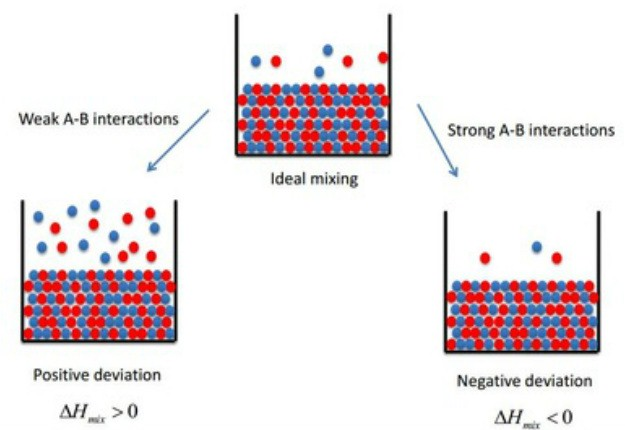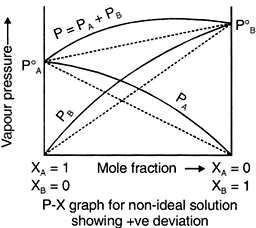> > Ideal and Non-ideal Solutions

# Ideal and Non-ideal Solutions

Too often, you would hear your parents telling you to be an ‘ideal’ kid. What do they mean by that? You better know that! But, when we say ideal and non-ideal solutions, what do we mean? In this chapter, we will study all about ideal and non-ideal solutions. We will look at their properties and examples.

### Suggested VideosIntroduction to Colligative PropertiesIntroduction to Abnormal Colligative PropertyNumericals on Elevation in Boiling Point## Raoult’s Law

In 1986, it was a French Chemist, Francois Marte Raoult who proposed a relationship between partial pressure and mole fraction of volatile liquids. According to the law, ‘the mole fraction of the solute component is directly proportional to its partial pressure’.On the basis of Raoult’s Law, liquid-liquid solutions can be of two types. They are:

• Ideal Solutions
• Non-ideal Solutions

## Ideal Solutions

The solutions which obey Raoult’s Law at every range of concentration and at all temperatures are Ideal Solutions. We can obtain ideal solutions by mixing two ideal components that are, solute and a solvent having similar molecular size and structure.

For Example, consider two liquids A and B, and mix them. The formed solution will experience several intermolecular forces of attractions inside it, which will be:

• A – A intermolecular forces of attraction
• B – B intermolecular forces of attraction
• A – B intermolecular forces of attraction

The solution is said to be an ideal solution, only when the intermolecular forces of attraction between A – A, B – B and A – B are nearly equal.

### Characteristics of Ideal Solutions

Ideal Solutions generally have characteristics as follows:

• They follow Raoult’s Law. This implies that the partial pressure of components A and B in a solution will be PA = PA0 xA and PB = PB0 xB .  PA0 and PB0 are respective vapour pressure in pure form. On the other hand, xA and xB are respective mole fractions of components A and B
• The enthalpy of mixing of two components should be zero, that is, Δmix H = 0. This signifies that no heat is released or absorbed during mixing of two pure components to form an ideal solution
• The volume of the mixing is equal to zero that is, Δmix V = 0. This means that total volume of solution is exactly same as the sum of the volume of solute and solution. Adding further, it also signifies that there will be contraction or expansion of the volume while the mixing of two components is taking place.
• The solute-solute interaction and solvent-solvent interaction is almost similar to the solute-solvent interaction.

### Examples of Ideal Solutions

• n-hexane and n-heptane
• Bromoethane and Chloroethane
• Benzene and Toluene
• CCl4 and SiCl4
• Chlorobenzene and Bromobenzene
• Ethyl Bromide and Ethyl Iodide
• n-Butyl Chloride and n-Butyl Bromide

## Non-Ideal Solutions

The solutions which don’t obey Raoult’s law at every range of concentration and at all temperatures are Non-Ideal Solutions. Non-ideal solutions deviate from ideal solutions and are also known as Non-Ideal Solutions.### Characteristics of Non-ideal Solutions

Non-ideal solutions depict characteristics as follows:

• The solute-solute and solvent-solvent interaction is different from that of solute-solvent interaction
• The enthalpy of mixing that is, Δmix H ≠ 0, which means that heat might have released if enthalpy of mixing is negative  (Δmix H < 0) or the heat might have observed if enthalpy of mixing is positive (Δmix H > 0)
• The volume of mixing that is,  Δmix V ≠ 0, which depicts that there will be some expansion or contraction in the dissolution of liquids

Non-ideal solutions are of two types:

• Non-ideal solutions showing positive deviation from Raoult’s Law
• Non-ideal solutions showing negative deviation from Raoult’s Law

### i) Positive Deviation from Raoult’s Law

Positive Deviation from Raoult’s Law occurs when the vapour pressure of the component is greater than what is expected in Raoult’s Law. For Example, consider two components A and B to form non-ideal solutions. Let the vapour pressure, pure vapour pressure and mole fraction of component A be PA , PA0 and xA respectively and that of component B be PB , PB0 and xB respectively. These liquids will show positive deviation when Raoult’s Law when:

• PA > PA0 xA and PB > P0B xB, as the total vapour pressure (PA0 xA + P0B xB) is greater than what it should be according to Raoult’s Law.
• The solute-solvent forces of attraction is weaker than solute-solute and solvent-solvent interaction that is, A – B < A – A or B – B
• The enthalpy of mixing is positive that is, Δmix H > 0 because the heat absorbed to form new molecular interaction is less than the heat released on breaking of original molecular interaction
• The volume of mixing is positive that is, Δmix V > 0 as the volume expands on the dissolution of components A and B

### Examples of Positive Deviation

Following are examples of solutions showing positive deviation from Raoult’s Law:

• Acetone and Carbon disulphide
• Acetone and Benzene
• Carbon Tetrachloride and Toluene or Chloroform
• Methyl Alcohol and Water
• Acetone and Ethanol
• Ethanol and Water

### Negative Deviation from Raoult’s Law

Negative Deviation occurs when the total vapour pressure is less than what it should be according to Raoult’s Law. Considering the same A and B components to form a non-ideal solution, it will show negative deviation from Raoult’s Law only when:

• PA < PA0 xA and PB < P0B xB as the total vapour pressure (PA0 xA + P0B xB) is less than what it should be with respect to Raoult’s Law
• The solute-solvent interaction is stronger than solute-solute and solvent-solvent interaction that is, A – B > A – A or B – B
• The enthalpy of mixing is negative that is, Δmix H < 0 because more heat is released when new molecular interactions are formed
• The volume of mixing is negative that is,  Δmix V < 0 as the volume decreases on the dissolution of components A and B

## A Solved Question for You

Q: Give some examples of solutions showing negative deviation from Raoult’s Law.

Solution: Following are examples of solutions showing negative deviation from Raoult’s Law

• Chloroform and Benzene
• Chloroform and Diether
• Acetone and Aniline
• Nitric Acid ( HNO3) and water
• Acetic Acid and pyridine
• Hydrochloric Acid ( HCl) and water

Share with friends

## Customize your course in 30 seconds

##### Which class are you in?
5th
6th
7th
8th
9th
10th
11th
12th
Get ready for all-new Live Classes!
Now learn Live with India's best teachers. Join courses with the best schedule and enjoy fun and interactive classes.Ashhar Firdausi
IIT Roorkee
BiologyDr. Nazma Shaik
VTU
ChemistryGaurav Tiwari
APJAKTU
Physics
Get StartedSubscribe
Notify of

## Question Mark?

Have a doubt at 3 am? Our experts are available 24x7. Connect with a tutor instantly and get your concepts cleared in less than 3 steps.